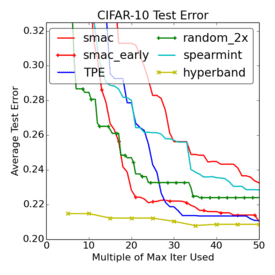This is part of our commercial offering.## V1Hyperband

``polyaxon.polyflow.matrix.hyperband.V1Hyperband(kind='hyperband', params=None, max_iterations=None, eta=None, resource=None, metric=None, resume=None, seed=None, concurrency=None, tuner=None, early_stopping=None)``

Hyperband is a relatively new method for tuning iterative algorithms. It performs random sampling and attempts to gain an edge by using time spent optimizing in the best way.

The algorithm tries a large number of random configurations/experiments, then decides which configurations to keep based on their progress.

The way Hyperband is implemented, it creates several buckets, each bucket has a number of randomly generated hyperparameter configurations, each configuration uses a resource (e.g. number of steps, number of epochs, batch size, …).

To adapt the algorithm’s maximum resource allocation, users can use `maxIterations`.

After trying a number of configurations, it chooses the top `number of observation/eta` configurations and runs them using an increased `resource*eta` resource. At last, it chooses the best configuration it has found so far.

The way Hyperband works is by discarding poor performing configurations leaving more resources for more promising configurations during the successive halving.

In order to use Hyperband correctly, you must define a metric called `resource` that the algorithm will increase iteratively.

• Args:
• kind: string, should be equal to `hyperband`
• params: List[Dict[str, params]]
• max_iterations: int
• eta: int
• resource: V1OptimizationResource
• metric: V1OptimizationMetric
• resume: bool, optional
• seed: int, optional
• concurrency: int, optional
• tuner: V1Tuner, optional
• early_stopping: List[EarlyStopping], optional

## YAML usage

``````matrix:
kind: hyperband
concurrency:
maxIterations:
resource:
metric:
resume:
params:
seed:
tuner:
earlyStopping:``````

## Python usage

``````from polyaxon import types
from polyaxon.polyflow import (
V1Hyperband, V1HpLogSpace, V1HpChoice, V1FailureEarlyStopping, V1MetricEarlyStopping,
V1OptimizationMetric, V1Optimization, V1OptimizationResource,
)
matrix = V1Hyperband(
concurrency=20,
params={"param1": V1HpLogSpace(...), "param2": V1HpChoice(...), ... },
resume=True,
metric=V1OptimizationMetric(name="loss", optimization=V1Optimization.MINIMIZE),
resource=V1OptimizationResource(name="num_steps", type=types.INT),
early_stopping=[V1FailureEarlyStopping(...), V1MetricEarlyStopping(...)]
)``````

## Fields

### kind

The kind signals to the CLI, client, and other tools that this matrix is hyperband.

If you are using the python client to create the mapping, this field is not required and is set by default.

``````matrix:
kind: hyperband``````

### params

A dictionary of `key -> value generator` to generate the parameters.

To learn about all possible params generators.

The parameters generated will be validated against the component’s inputs/outputs definition to check that the values can be passed and have valid types.

``````matrix:
kind: hyperband
params:
param1:
kind: ...
value: ...
param2:
kind: ...
value: ...``````

### maxIterations

The algorithm’s maximum resource allocation.

``````matrix:
kind: hyperband
maxIterations: 81``````

### eta

A parameter that tunes:

• The downsampling factor: `number of observation/eta`
• The resource increase factor: `resource*eta`
``````matrix:
kind: hyperband
eta: 3``````

### resource

The resource to optimize (should be an int or a float), the resource van be the number of steps or epochs,

``````matrix:
kind: hyperband
resource:
name: num_steps
type: int``````

### metric

The metric to optimize during the iterations, this is the metric that you want to maximize or minimize.

``````matrix:
kind: hyperband
metric:
name: loss
optimization: minimize``````

### resume

A flag to resume or restart the selected runs, default to false (restart)

``````matrix:
kind: hyperband
resume: True``````

### concurrency

An optional value to set the number of concurrent operations.

This value only makes sense if less or equal to the total number of possible runs.
``````matrix:
kind: random
concurrency: 2``````

### seed

Since this algorithm uses random generators, if you want to control the seed for the random generator, you can pass a seed.

``````matrix:
kind: hyperband
seed: 523``````

### earlyStopping

A list of early stopping conditions to check for terminating all operations managed by the pipeline. If one of the early stopping conditions is met, a signal will be sent to terminate all running and pending operations.

``````matrix:
kind: hyperband
earlyStopping: ...``````

For more details please check the early stopping section.

### tuner

The tuner reference (w/o a component hub reference) to use. The component contains the logic for creating new suggestions, users can override this section to provide a different tuner component.

``````matrix:
kind: hyperband
tuner:
hubRef: 'acme/my-hyperband-tuner:version'``````

## Example

This is an example of using hyperband for hyperparameter search:

``````version: 1.1
kind: operation
matrix:
kind: hyperband
concurrency: 5
maxIterations: 81
eta: 3
resource:
name: num_steps
type: int
metric:
name: loss
optimization: minimize
resume: False
params:
lr:
kind: uniform
value: [0, 0.9]
dropout:
kind: choice
value: [0.25, 0.3]
activation:
kind: pchoice
value: [[relu, 0.1], [sigmoid, 0.8]]
early_stopping:
- metric: accuracy
value: 0.9
optimization: maximize
- metric: loss
value: 0.05
optimization: minimize
component:
inputs:
- name: batch_size
type: int
isOptional: true
value: 128
- name: logspace
type: float
- name: dropout
type: float
container:
image: image:latest
command: [python3, train.py]
args: [
"--batch-size={{ batch_size }}",
"--lr={{ lr }}",
"--dropout={{ dropout }}",
"--activation={{ activation }}"
]``````

In this example we allocate a maximum resources of `81`, our resource in this case is the `num_steps` which is of type `int` that we pass to our model.

This is how the algorithm works with this config:

bucket=4 bucket=3 bucket=2 bucket=1 bucket=0
iteration num configs resource alloc num configs resource alloc num configs resource alloc num configs resource alloc num configs resource alloc
0 81 1 27 3 9 9 6 27 5 81
1 27 3 9 9 3 27 2 81
2 9 9 3 27 1 81
3 3 27 1 81
4 1 81